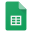# Count cells greater than in Excel and Google Sheets

=COUNTIF(A:A,">10")

=COUNTIF(A:A,">"&B3) // Criteria as a cell reference

### A:A = Range

Check below for a detailed explanation with pictures and how to use formulas in Excel and Google Sheets.

# Count cells greater than in Excel

How to count cells greater than in Excel?# COUNT CELLS GREATER THAN — EXCEL FORMULA AND EXAMPLE

1. =COUNTIF(A:A,">10")

• Criteria within the formula and must be inside the quotation mark

2. =COUNTIF(A:A,">"&B3)

• Condition within the formula and must be inside the quotation mark

• Criteria as a cell reference

3. =COUNTIF(A:A,B4)

• Criteria as a cell reference

4. =COUNTIF(A:A,B5)

• Criteria as a cell reference

# Count cells greater than in Google Sheets

How to count cells greater than in Google Sheets?# COUNT CELLS GREATER THAN — GOOGLE SHEETS FORMULA AND EXAMPLE

1. =COUNTIF(A:A,">10")

• Criteria within the formula and must be inside the quotation mark

2. =COUNTIF(A:A,">"&B3)

• Condition within the formula and must be inside the quotation mark

• Criteria as a cell reference

3. =COUNTIF(A:A,B4)

• Criteria as a cell reference

4. =COUNTIF(A:A,B5)

• Criteria as a cell reference

## Wild cards and regular expressions in Excel and Google SheetsWild cards and regular expressions